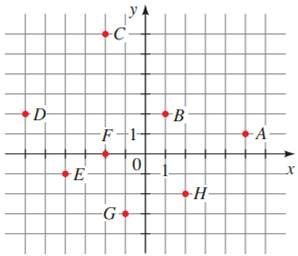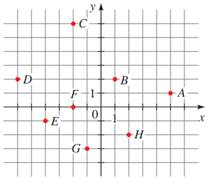# The coordinates of the points from the figure provided below,### Precalculus: Mathematics for Calcu...

6th Edition
Stewart + 5 others
Publisher: Cengage Learning
ISBN: 9780840068071### Precalculus: Mathematics for Calcu...

6th Edition
Stewart + 5 others
Publisher: Cengage Learning
ISBN: 9780840068071

#### Solutions

Chapter 1.8, Problem 8E
To determine

## To calculate: The coordinates of the points from the figure provided below,Expert Solution

The coordinates of the points are,

PointCoordinateA(5,1)B(1,2)C(2,6)D(6,2)E(4,1)F(2,0)G(1,3)H(2,2)

### Explanation of Solution

Given information:

The points are marked in the Cartesian planeFormula used:

The Cartesian plane is divided into four quadrant. Numbers on right of zero are positive, on left of zero are negative. Numbers above zero on vertical line are positive and numbers below 0 are negative. Each pair of point in the coordinate plane is represented as an ordered pair (x,y) where first element is the x-coordinate and second element is the y-coordinate. The point (x,y) denote it is x units on x-axis and y units on y −axis and marked accordingly.

Calculation:

Consider the provided points that are marked in the Cartesian planeObserve that the Cartesian plane is divided into four quadrant. Numbers on right of zero are positive, on left of zero are negative. Numbers above zero on vertical line are positive and numbers below 0 are negative. Each pair of point in the coordinate plane is represented as an ordered pair (x,y) where first element is the x-coordinate and second element is the y-coordinate. The point (x,y) denote it is x units on x-axis and y units on y −axis and marked accordingly.

For the point A , x-coordinate is 5 and y-coordinate is 1.

For the point B , x-coordinate is 1 and y-coordinate is 2.

For the point C , x-coordinate is 2 and y-coordinate is 6.

For the point D , x-coordinate is 6 and y-coordinate is 2.

For the point E , x-coordinate is 4 and y-coordinate is 1 .

For the point F , x-coordinate is 2 and y-coordinate is 1.

For the point G , x-coordinate is 1 and y-coordinate is 3 .

For the point H , x-coordinate is 2 and y-coordinate is 2 .

Therefore, the coordinates of points is tabulated as,

PointCoordinateA(5,1)B(1,2)C(2,6)D(6,2)E(4,1)F(2,0)G(1,3)H(2,2)

### Have a homework question?

Subscribe to bartleby learn! Ask subject matter experts 30 homework questions each month. Plus, you’ll have access to millions of step-by-step textbook answers!